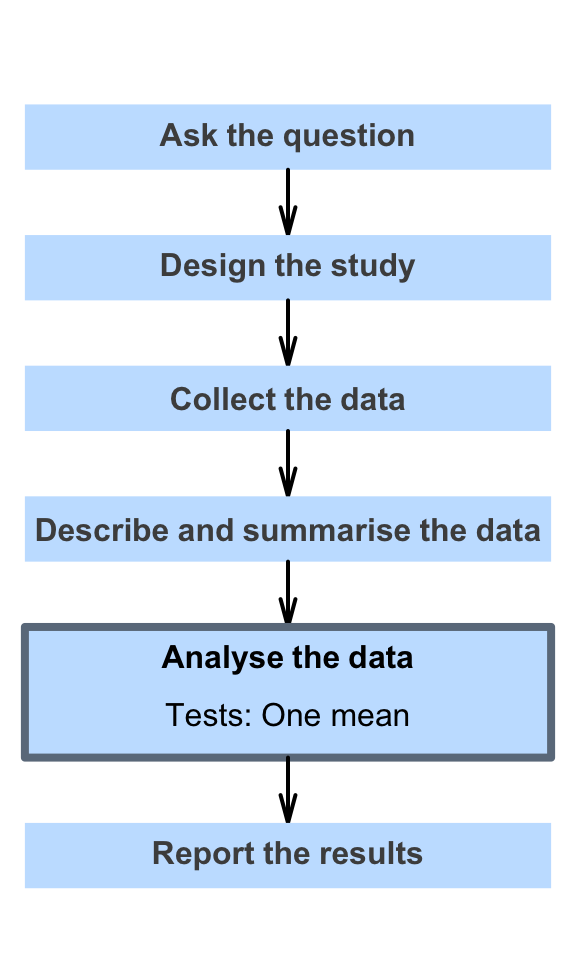# 27 Hypothesis tests for one mean

So far, you have learnt to ask a RQ, identify different ways of obtaining data, design the study, collect the data describe the data, summarise data graphically and numerically, understand the tools of inference, and to form confidence intervals.

In this chapter, you will learn about hypothesis tests for one mean. You will learn to:

• conduct hypothesis tests for one sample mean, using a $$t$$-test.
• determine whether the conditions for using these methods apply in a given situation.